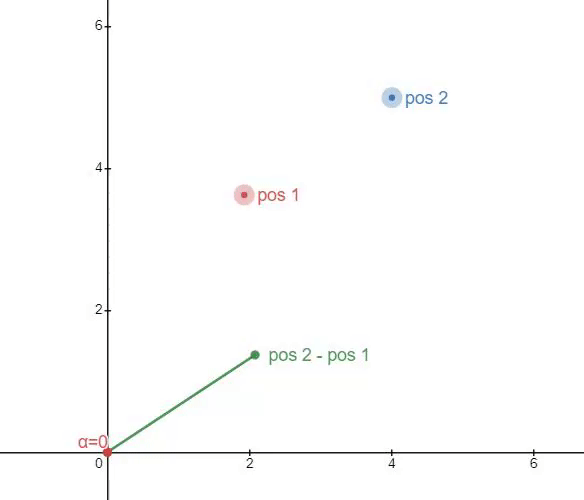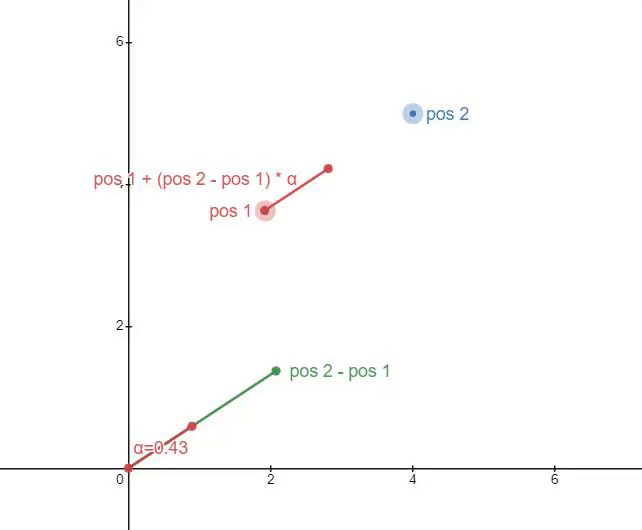# Help on Finding a Position Between Two Parts

I’m trying to find a position between two parts based on a percentage. For example, given the positions (10,0,10) and (2,0,0), and the percentage 25%, I want the script to return the position (4,0,2.5)

If one of the points aren’t at the origin (0,0,0) then the system breaks.

I have looked for around 30 minutes on YouTube/Developer Hub, and have found nothing.

``````local percentage = 25

while wait() do
newPercentage = percentage/100

part1 = game.Workspace.Part1
part2 = game.Workspace.Part2

movePart = game.Workspace.MovePart

newPosition = (part1.Position+part2.Position)*newPercentage

movePart.Position = Vector3.new(newPosition.X,0.5,newPosition.Z)

end
``````
1 Like

1 Like

Just like nube said above, theres actually a built in function for this, however, if you do want to code it yourself though youd do something like

``````pos1+(pos2-pos1)*percentage
``````

The reason it only worked at the origin is because youre basically just “dividing” the existing value according to the origin since you forgot to put it relative to pos1 by subtracting it
The complete answer actually uses a similar principle, because it looks at pos2 as if it were relative to the origin by subtracting pos1 from it, then it readds it, as shown below:

First step, move it down to the origin
Then you multiply it by a value from 0 to 1 to modify its length(α is the fancy greek letter for the percentage)

The third and final step is to just move this new alpha value back up to pos1Hopefully this brings some clarity to the original problem, just in case you were curious about exactly what went wrong and exactly what you should do

1 Like